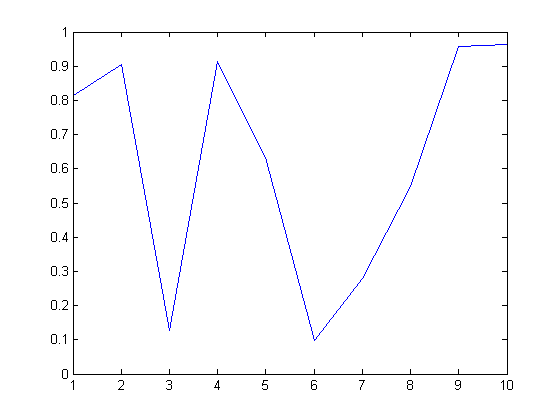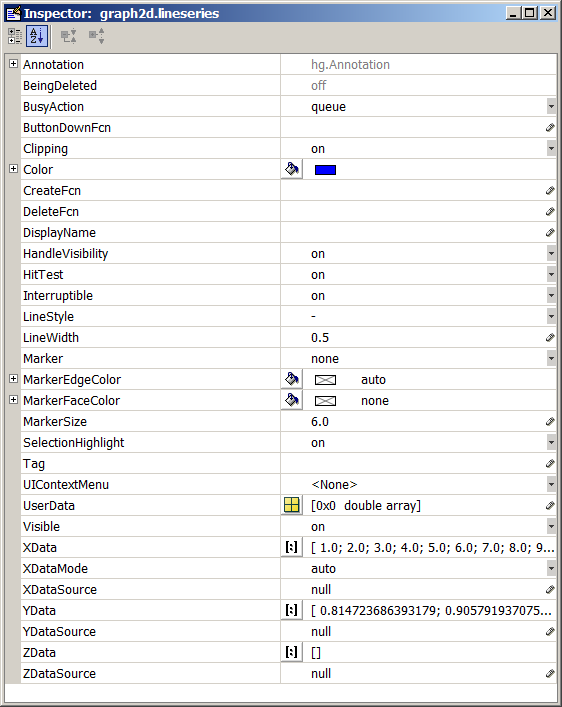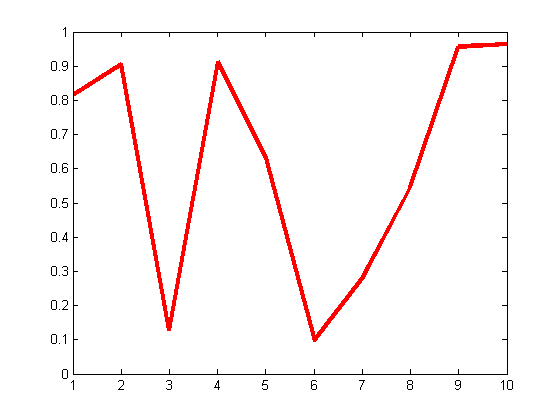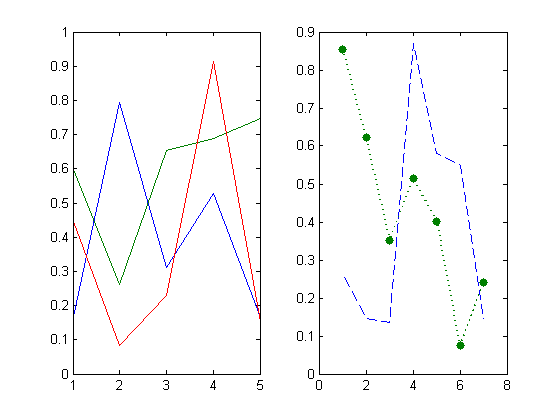# Handle Graphics

Note: You can execute the code from this tutorial by highlighting them, right-clicking, and selecting "Evaluate Selection" (or hit F9).

Handle Graphics is concept that MATLAB uses to deal with all MATLAB graphics. By understanding the concept of Handle Graphics, you will be able to customize just about anything graphics related.

## Getting a Handle to a Graphics Object

Almost all plotting functions return a handle to the graphics object. For example, if you call plot with an output argument,

```x = rand(10, 1);
h = plot(x)
```
```h =
176.0028
```you get a "handle" to the line that was plotted. h may just look like a number (double precision), but internally MATLAB interprets it as a handle (or a pointer) to the line object.

Give it a Try

Try plotting multiple lines. How many handles do you get back?

` h2 = plot(rand(10, 3))`

## Inspecting Graphics Properties (Interactively)

Now that you have a handle to the graphics object, what can you do with it? You can use the handle to access all the properties associated with the object. You can look at it and change it. To get a view of the current property settings, you can do it interactively.

` inspect(h)`Changing the properties with the Property Inspector will reflect the change immediately.

Give it a Try

Try changing some of the properties and see the effect.

## Accessing Graphics Properties (Programmatically)

You can also access graphics properties programmatically using the get and set commands.

```get(h)
```
```           DisplayName: ''
Annotation: [1x1 hg.Annotation]
Color: [0 0 1]
LineStyle: '-'
LineWidth: 0.5000
Marker: 'none'
MarkerSize: 6
MarkerEdgeColor: 'auto'
MarkerFaceColor: 'none'
XData: [1 2 3 4 5 6 7 8 9 10]
YData: [1x10 double]
ZData: [1x0 double]
BeingDeleted: 'off'
ButtonDownFcn: []
Children: [0x1 double]
Clipping: 'on'
CreateFcn: []
DeleteFcn: []
BusyAction: 'queue'
HandleVisibility: 'on'
HitTest: 'on'
Interruptible: 'on'
Selected: 'off'
SelectionHighlight: 'on'
Tag: ''
Type: 'line'
UserData: []
Visible: 'on'
Parent: 175.0023
XDataMode: 'auto'
XDataSource: ''
YDataSource: ''
ZDataSource: ''
```

If you want to change the color of the line to read and the line thickness to 3

```set(h, 'Color', 'red', 'LineWidth', 3)
```Getting the Underlying Data

MATLAB plots contain the underlying data as well, so you can extract the data directly from their handles:

```get(h, 'XData')
get(h, 'YData')
```
```ans =
Columns 1 through 9
1     2     3     4     5     6     7     8     9
Column 10
10
ans =
Columns 1 through 5
0.8147    0.9058    0.1270    0.9134    0.6324
Columns 6 through 10
0.0975    0.2785    0.5469    0.9575    0.9649
```

## Type of Graphics Object

In addition to knowing that each graphics object has a set of properties, it's important to know that there are different types of graphic objects. You can get that by the type property:

```get(h, 'Type')
```
```ans =
line
```

## Handle Graphics Hierarchy

What are the different types of graphics objects and how are they related?

An important concept to understand is the Handle Graphics Hierarchy. Only certain types graphics objects can reside within a particular object. For example, a line object can be placed inside an axes object, but not inside a figure object. That means that, in order to plot a line, there must also be an axes. Conveniently, when you try to plot a line, MATLAB takes care of that by creating new axes if one does not exist.

This hierarchy is represented with a parent-children concept. An object can only have one parent, while a parent can have multiple children. For example, we can get the handle to the parent object for the line:

```ph = get(h, 'Parent')
```
```ph =
175.0023
```

And we can see the properties of the parent object:

```get(ph)
```
```	ActivePositionProperty = outerposition
ALim = [0 1]
ALimMode = auto
AmbientLightColor = [1 1 1]
Box = on
CameraPosition = [5.5 0.5 17.3205]
CameraPositionMode = auto
CameraTarget = [5.5 0.5 0]
CameraTargetMode = auto
CameraUpVector = [0 1 0]
CameraUpVectorMode = auto
CameraViewAngle = [6.60861]
CameraViewAngleMode = auto
CLim = [0 1]
CLimMode = auto
Color = [1 1 1]
CurrentPoint = [ (2 by 3) double array]
ColorOrder = [ (7 by 3) double array]
DataAspectRatio = [9 1 2]
DataAspectRatioMode = auto
DrawMode = normal
FontAngle = normal
FontName = Helvetica
FontSize = 
FontUnits = points
FontWeight = normal
GridLineStyle = :
Layer = bottom
LineStyleOrder = -
LineWidth = [0.5]
MinorGridLineStyle = :
NextPlot = replace
OuterPosition = [0 0 1 1]
PlotBoxAspectRatio = [1 1 1]
PlotBoxAspectRatioMode = auto
Projection = orthographic
Position = [0.13 0.11 0.775 0.815]
TickLength = [0.01 0.025]
TickDir = in
TickDirMode = auto
TightInset = [0.0392857 0.0404762 0.0142857 0.0190476]
Title = [180.002]
Units = normalized
View = [0 90]
XColor = [0 0 0]
XDir = normal
XGrid = off
XLabel = [177.002]
XAxisLocation = bottom
XLim = [1 10]
XLimMode = auto
XMinorGrid = off
XMinorTick = off
XScale = linear
XTick = [ (1 by 10) double array]
XTickLabel =
1
2
3
4
5
6
7
8
9
10
XTickLabelMode = auto
XTickMode = auto
YColor = [0 0 0]
YDir = normal
YGrid = off
YLabel = [178.002]
YAxisLocation = left
YLim = [0 1]
YLimMode = auto
YMinorGrid = off
YMinorTick = off
YScale = linear
YTick = [ (1 by 11) double array]
YTickLabel = [ (11 by 3) char array]
YTickLabelMode = auto
YTickMode = auto
ZColor = [0 0 0]
ZDir = normal
ZGrid = off
ZLabel = [179.002]
ZLim = [-1 1]
ZLimMode = auto
ZMinorGrid = off
ZMinorTick = off
ZScale = linear
ZTick = [-1 0 1]
ZTickLabel =
ZTickLabelMode = auto
ZTickMode = auto

BeingDeleted = off
ButtonDownFcn =
Children = [176.003]
Clipping = on
CreateFcn =
DeleteFcn =
BusyAction = queue
HandleVisibility = on
HitTest = on
Interruptible = on
Parent = [174.002]
Selected = off
SelectionHighlight = on
Tag =
Type = axes
UserData = []
Visible = on

```

With this technique, you can pretty much access any graphics objects by traversing up and down the hierarchy.

## Finding Handles

What if I don't have a handle to work with? For example, if I happen to call the plotting commands without any output arguments. Or if I just open a FIG file:

```openfig('Fig1.fig')
```There are a number of techniques you can use to get the handles of existing graphic objects:

• gcf - handle of the current figure. MATLAB has a concept of a "current" figure.
• gca - handle of the current axes.
• gco - handle of the current object. This returns the handle of the last clicked object. The object could be anything, including axes and figures.
• findobj - find objects with specific properties.
• findall - find objects (including hidden objects).

Give it a Try

1. Open "Fig1.fig".
2. Click on the green line with dots (on the right axes) and use gco to get the handle of the line object. Access and change some of the properties of the line.
3. Find all lines with color blue:
`     h = findobj('Type', 'line', 'Color', 'blue')`